In :
%matplotlib inline
import matplotlib.pyplot as plt
import numpy as np

Recall the "normal" probability distribution: $$\qquad\qquad{\rm gaussian}(x)=\frac{1}{\sigma\sqrt{2\pi}}{\rm e}^{\textstyle-\frac{(x-\mu)^2}{2\sigma^2}}$$

In :
def gaussian(x,mu=0.,sigma=1.):
return np.exp(-(x-mu)**2/(2.*sigma**2))/(sigma*np.sqrt(2.*np.pi))
In :
x=np.arange(-5,5.1,.1)
y=gaussian(x)
plt.plot(x,y)
plt.xticks(range(-4,5))
plt.xlim(-4,4);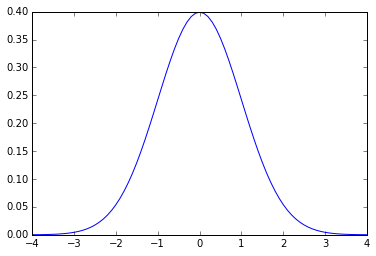Since it's a probability distribution, the total integral under the curve is 1; and since it's symmetric about the origin the area under left and right halves is .5. To get a rough feeling for various fractions of the area under the curve, we can approximate the integral by doing a sum, with stepsize of .0001. To get the area up to any given xmax, we can integrate from 0 to xmax and then add .5 for the left half (rather than sum all the way from $-\infty$ to xmax):

In :
# "cumulative distribution function"
def cdf(xmax): return .0001*sum(gaussian(np.arange(-6,xmax,.0001)))
In :
for sigma in range(-4,5): print (sigma, round(cdf(sigma),5))
-4 3e-05
-3 0.00135
-2 0.02275
-1 0.15866
0 0.5
1 0.84134
2 0.97725
3 0.99865
4 0.99997

So the area up to $x=1$ is roughly 84%, and increases to almost 100% for $x=4$. To get the values between some $\pm x$, we can use cdf(x) - cdf(-x), as depicted below:

In :
# see https://www.inferentialthinking.com/chapters/12/3/sd-and-the-normal-curve.html
plt.figure(figsize=(12,7))
x=np.arange(-5,5.1,.1)
y=gaussian(x)

def gfig(xt,yt,t,t0,sb):
plt.subplot(2,2,sb)
plt.plot(x,y,'k')
plt.gca().set_axisbelow(True)
plt.yticks(np.arange(0,.5,.1))
plt.grid('on')
plt.axis([-4,4,0,.45])
plt.text(xt,yt,t,fontsize=15)
plt.text(-3.5,.35,t0,fontsize=14)

gfig(-.75,.15,.841,'cdf(1)',1)
plt.fill_between(x,y,color='gold',where=x<1.01)

gfig(1.5,.15,.159,'1 - cdf(1)',2)
plt.fill_between(x,y,color='gold',where=x>.99)

gfig(-.6,.15,.683,'cdf(1) - cdf(-1)',3)
plt.fill_between(x,y,color='gold',where=x<1.01)
plt.fill_between(x,y,color='darkblue',where=x<-.99)

gfig(-.5,.15,.954,'cdf(2) - cdf(-2)',4)
plt.fill_between(x,y,color='gold',where=x<2.01)
plt.fill_between(x,y,color='darkblue',where=x<-1.99)
None;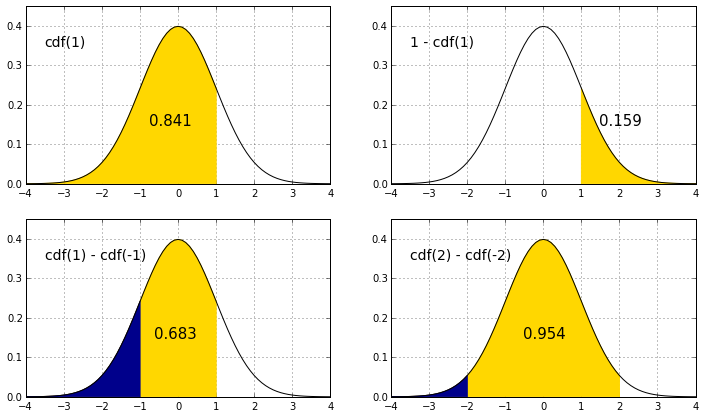(For values between $\pm x$ we could also have used 2*(cdf(x) - cdf(0))= 2*(cdf(x)-.5), which gives the same result by doubling the area under the curve to the right of 0).

The fractional values for +/- 1,2,3 standard deviations $\sigma$ from the mean reproduce the "68–95–99.7" rule:

In :
for sigma in range(1,5):
print (sigma,round(cdf(sigma)-cdf(-sigma),5))
(1, 0.68269)
(2, 0.9545)
(3, 0.9973)
(4, 0.99994)

(Note that the value for $\sigma=2$ rounds to .95 — the more accurate value for an exact .95 confidence interval is closer to $\sigma=1.96$.)

The above values are twice the "cumulative from mean", which include only the probability starting at the mean (rather than both above and below).

Those latter are tabulated in terms of "z-values", as found in standard tables. The "z-value" is defined as the (value - mean)/std , a simple rescaling that permits viewing any normal distribution interms of a corresponding distribution with mean = 0, and std = 1.

In :
plt.figure(figsize=(8,4))
x=np.arange(-5,5.1,.1)
y=gaussian(x)
plt.plot(x,y)
plt.fill_between(x,y,color='blue',where=abs(x)<3.01)
plt.fill_between(x,y,color='#00AAAA',where=abs(x)<2.01)
plt.fill_between(x,y,color='cyan',where=abs(x)<1.01)
for i,iy,pct in (1,.235,' 68'),(2,.05,' 95'),(3,.02,'99.7'):
plt.annotate(pct+'%',xytext=(i+(.4 if i < 3 else .46),iy),xy=(.4,iy),
arrowprops={'arrowstyle':"<-"},ha='center',va='center',fontsize=14)
plt.annotate('$\pm'+str(i)+'\sigma$',xy=(-(i+.05),iy),xytext=(0,iy),
arrowprops={'arrowstyle':"->"},ha='center',va='center',fontsize=15)
plt.xticks(range(-4,5))
plt.xlim(-4,4);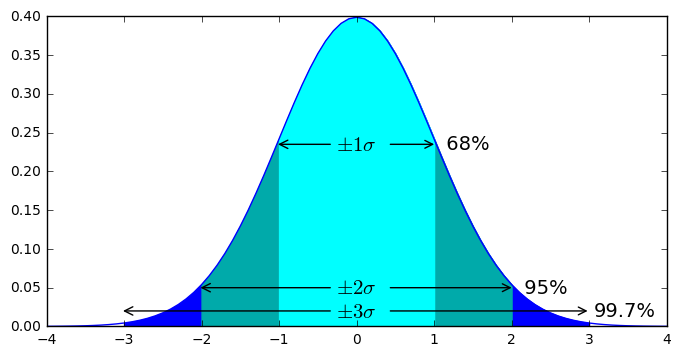As described above, the values 1 - cdf(xmax) give the area under the curve for x > xmax:

In :
[(sigma,round(1-cdf(sigma),5)) for sigma in range(1,5)]
Out:
[(1, 0.15865), (2, 0.02273), (3, 0.00133), (4, 1e-05)]
We see that values of x more than roughly 1.645 standard deviations above the mean correspond to the typically used p-value of .05:
In :
round(1-cdf(1.645),4)
Out:
0.05
In :
x=np.arange(-5,5.1,.05)
y=gaussian(x)
plt.plot(x,y)
plt.fill_between(x,y,color='r',where=x>1.64)
plt.annotate('$1.645\sigma$',xytext=(.5,.08),xy=(1.645,.08),
arrowprops=dict(arrowstyle="->"),ha='center',va='center',fontsize=15)
plt.annotate('5%',xytext=(2.5,.08),xy=(2,.02),
arrowprops=dict(arrowstyle="->"),ha='center',va='center',fontsize=14)
plt.xticks(np.arange(-4,5));
plt.xlim(-4,4);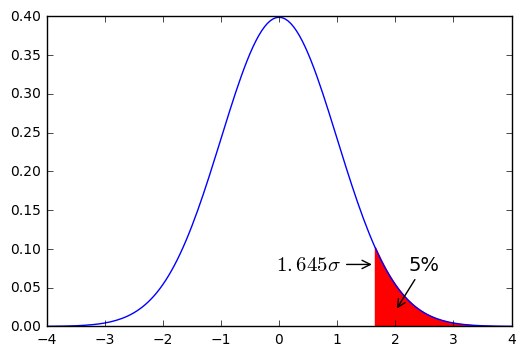These values can also be obtained from "z-values" tabulated in standard tables mentioned above.

The "cumulative z-value" gives the probability that a statistic is less than z (given here by the cdf for a normal distribution with mean 0 and std 1), and the probability of being above that value is called the "complementary cumulative", equal to 1 minus the cumulative z-value.
(So the cumulative z-value of 1.645 is roughly .95, and the complementary cumulative z-value is .05 .)

In [ ]:

Finally, a quick look at the Central limit theorem: consider a set of $N$ independent random variables $X_i$, with arbitrary probability distributions $p_i(x)$, with means $\mu_i$ and variances $\sigma_i^2$, If we consider the sum $X=\sum_{i=1}^N X_i$, then for sufficiently large $N$, and whatever the other properties of those independent probability distributions, the probability of $X$ will tend to a gaussian (normal) distribution with $\mu=\sum_{i=1}^N \mu_i$ and variance $\sigma^2=\sum_{i=1}^N\sigma_i^2$.

[From here, until the colorblindness example, was touched on briefly but not discussed in detail in class]

As an illustration of the central limit theorem, we'll consider a set of $N=100$ biased coins, given by the following array of probabilities for flipping heads:

In :
N=100
from numpy.random import rand
ps=rand(N)
#generates array of N random numbers valued between 0 and 1
print ps
m=sum(ps)
s=np.sqrt(sum(ps*(1-ps)))
print '\n sum = ',m,', sqrt of sum of variances=',s
[ 0.58504838  0.06749052  0.67847329  0.02145685  0.8115801   0.00972488
0.98008872  0.16741078  0.32827107  0.29629761  0.47004204  0.46377653
0.29797421  0.53004542  0.57496569  0.85419513  0.16823474  0.2411057
0.56718669  0.44564699  0.6471145   0.41155465  0.84302772  0.31185329
0.56828312  0.71480349  0.30532747  0.49263149  0.55954655  0.63535911
0.32994111  0.39422062  0.43126911  0.07669763  0.37744275  0.31518262
0.02127374  0.49129903  0.94020867  0.8701907   0.18943227  0.25165163
0.36721112  0.8646983   0.01688388  0.68209579  0.54719819  0.21998912
0.88638086  0.02147168  0.05109274  0.87075567  0.83089441  0.72786461
0.16501426  0.38768371  0.81621628  0.01167828  0.72784704  0.51255012
0.48597073  0.91955029  0.49351834  0.55223251  0.87815811  0.64242302
0.89705262  0.08686844  0.15739173  0.72662814  0.66653257  0.54222933
0.01396583  0.76119011  0.34971616  0.60344439  0.82488714  0.46769399
0.53797511  0.35909236  0.34092861  0.1409124   0.18336853  0.46919407
0.89969404  0.99563876  0.77763804  0.2285255   0.60089223  0.28648359
0.96840626  0.32233254  0.34364086  0.38650164  0.06723778  0.68044252
0.8808488   0.37315962  0.1524534   0.94894805]

sum =  48.4586208535 , sqrt of sum of variances= 4.13107780047
In :
S = 100000 # number of simulations
# count the number of times the N random numbers are less than the corresponding entry in ps (# successes):
def rtrials(): return sum(rand(N) < ps)
results = [rtrials() for t in xrange(S)]
print np.mean(results),np.std(results)
48.46257 4.14122433528

We see these compare well to the above values expected from the central limit theorem, and the probability distribution for $X$ is gaussian, even though it is composed from the sum of one hundred different (and non-gaussian) random variables:

In :
plt.figure(figsize=(8,4))
plt.hist(results,np.arange(-.5,101),label='data');
xg=np.arange(int(m-4*s)+1,int(m+4*s),.1)
yg = gaussian(xg,m,s)
plt.plot(xg,S*yg,'r-',label='gaussian',linewidth=1.5)
plt.plot((m,m),(0,S*gaussian(m,m,s)),'y--')
plt.annotate('',xytext=(m-s,S*gaussian(m-s,m,s)),xy=(m+s,S*gaussian(m+s,m,s)),
arrowprops=dict(arrowstyle="<->"))
plt.title('data fit by gaussian with predicted mean={}, stdev={}'.format(round(m,1),round(s,1)))
plt.xlim(int(m-4*s)+1,int(m+4*s));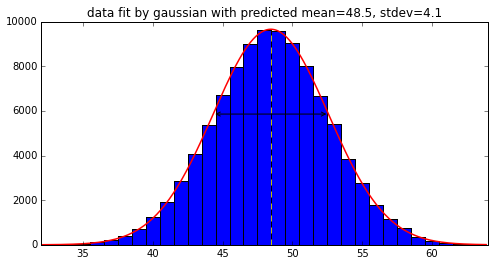Note that N needn't even be as large as 100 for the central limit theorem to work reasonably well -- retry the above, e.g., for N=30.

For another example of arbitrary probability distributions combining to form a normal distribution, consider a population with bimodal heights: half this population has height of exactly 5.5 feet, and the other half has height of exactly 6 feet. We choose N people at random and measure the average of their heights. That average will be normally distributed, with a mean of 5.75 and a standard deviation of sqrt(N/16.)/N $=1/4\sqrt N$.
(1/16. is the variance of the above distribution, since each of the possibilities is 1/4. from the mean, and the additional factor of N in the denominator is because we are considering the mean rather than the sum.) For N=100, the std is 1/40. = .025 .

In :
def height(n):
return [6 if tall else 5.5 for tall in rand(n) > .5]
In :
N=100
S=100000 # number of simulations
results = [np.mean(height(N)) for t in xrange(S)]
In :
print np.mean(results),'expected mean',5.75
print np.std(results),'expected std',1/40.
5.74999825 expected mean 5.75
0.0249461970436 expected std 0.025

We see that the mean and std of the distribution are as expected, and in addition the full probability distribution is well described by a normal distribution (where the average heights over 100 people can take fractional values in between 5.5 and 6 even though any individual height is one of those two extremes with equal probability):

In :
step=.005
plt.hist(results,np.arange(5.5-step/2,6.1,step),label='data');
xg=np.arange(5.6,6,step/5)
yg=step*S*gaussian(xg,5.75,1./40)
plt.plot(xg,yg,'r')
plt.xlabel('average height of '+str(N)+' bimodal people')
plt.xlim(5.65,5.85);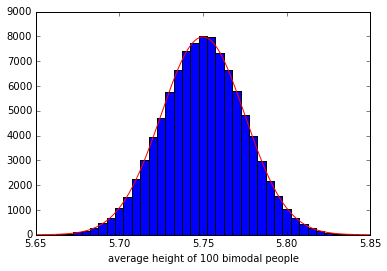In [ ]:

Color blindness example¶

As a last example, consider measuring samples of the population for some trait. Standard red/green color blindness affects roughly 8% of males (the relevant gene that codes for the pigment in the retinal cone cells is on the X chromosome, so is sex-linked, and only .6% of females are affected since that would require two of the variant X chromosomes, .08*.08=.0064). Suppose that 194 boys in an incoming class of high school students are tested for color blindness, what range of results is expected?

We're considering drawing the sample at random from some large population, so the result of the test can be considered a random variable with a probability of .08 of testing positive. For a set of n samples with probability p, define:

In :
# from http://colormax.org/color-blind-test/ , should see a green digit
from IPython.display import Image
Out: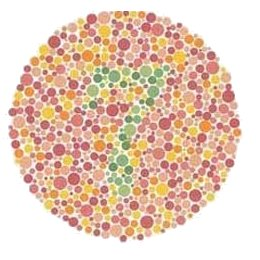In :
def rn(p,n): return sum(rand(n) <= p)

This will return the total number of n that test positive, if each has a probability of p of testing positive.

These results should be roughly normally distributed, so if we do some large number of simulations:

In :
S=100000 # number of simulations
results = [rn(.08,194) for t in xrange(S)]
In :
print np.mean(results),'expected',.08*194
print np.std(results),'expected',np.sqrt(.08*.92*194)
15.5197 expected 15.52
3.7617857342 expected 3.77867701716

We see that the distribution is roughly a normal distribution:

In :
plt.hist(results,bins=np.arange(-.5,36))
xg=np.arange(0.,35.,.1)
yg=S*gaussian(xg,.08*194,np.sqrt(.08*.92*194))
plt.plot(xg,yg,'r-',linewidth=2)
xr=np.arange(15.526-4*3.771,15.526+5*3.771,3.771)
plt.vlines(xr,0,S/40,'m',linewidth=2);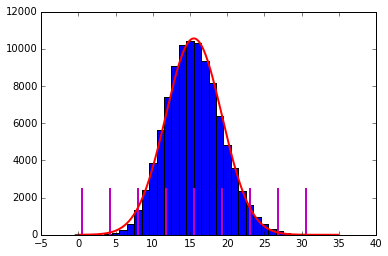where the expected mean, and $\pm 1,2,3$ standard deviations are indicated by magenta lines.
From the '68-95-99.7' rule, it's very likely (95%) that the results of a single measurement will fall between $15.52 \pm 2\cdot 3.78$, so from 8 to 23, and almost certain (99.7%) that they'll fall between $15.52 \pm 3\cdot 3.78$, so from 4 to 27 measured to have color blindness.

Standard preprogrammed functions¶

There are a number of different preprogrammed ways to draw from normal distributions with specified mean and standard deviation, including random.normalvariate(), numpy.random.normal(), and scipy.stats.norm.rvs(). Here we'll standardize on the scipy.stats.norm module, which has the following:

In :
from scipy.stats import norm
#http://docs.scipy.org/doc/scipy-0.14.0/reference/generated/scipy.stats.norm.html
#has some other useful methods, e.g.
#rvs random variates
#pdf prob density function: same as the exp(-...) in gaussian() above
#cdf cumulative density function: the integral under curve from -infinity to x (q(x)/2 + 1/2)
#ppf percent point function -- inverse of cdf, to get x for given pct
In :
print 'five drawn from mean=0, std=1:',norm.rvs(0,1,5)
print 'five drawn from mean=100, std=20:',norm.rvs(100,20,5)
five drawn from mean=0, std=1: [ 1.2352734   0.09930125 -1.2617659  -0.58465427  0.80584166]
five drawn from mean=100, std=20: [ 105.93527007  101.64849761  135.96842401  102.12431093   99.59081446]
In :
S=1000 #number of random draws
xdata=np.arange(-4,4,.1)
plt.plot(xdata,S*.1*gaussian(xdata),'r')
plt.hist(norm.rvs(0,1,S),bins=xdata);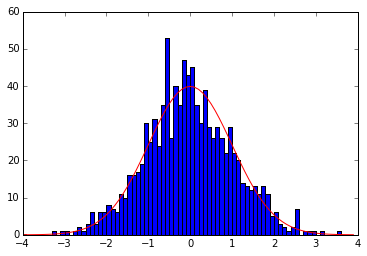Note in particular that the norm.cdf() function is a more accurate version of the simple cumulative distribution function defined above:

In :
print 'p=.05:',1-norm.cdf(1.645)
print '[68, 95, 99.7]:',[2*norm.cdf(sigma) - 1 for sigma in 1,2,3]
p=.05: 0.0499849055391
[68, 95, 99.7]: [0.68268949213708585, 0.95449973610364158, 0.99730020393673979]

And norm.pdf() is the probability distribution function, i.e., the gaussian() function defined above:

In :
x = np.linspace(-3, 3, 101)
plt.plot(x,norm.pdf(x));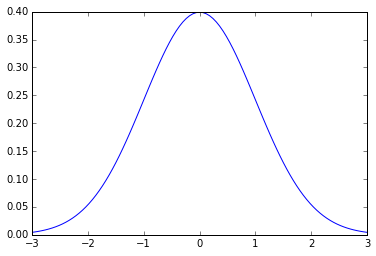Recall the cdf gives the probability of all values from $-\infty$ up to some number of standard deviations. norm.ppf(), the "percent point function", is the inverse of that, i.e., for a given value of probability, it gives the corresponding number of standard deviations (below which down to $-\infty$ have that probability).

In :
print norm.ppf(0.05),norm.ppf(0.95) #get std dev for p=.05, 90% within
print norm.ppf(.1), norm.ppf(.9) # 80% within boundaries
-1.64485362695 1.64485362695
-1.28155156554 1.28155156554
In :
#again find std corresponding to 68-95-99.7 rule, and the 90 value (p=.05 above)
for p in (.68,.9,.95,.997):
print p,.5+p/2,norm.ppf(.5+p/2)
0.68 0.84 0.99445788321
0.9 0.95 1.64485362695
0.95 0.975 1.95996398454
0.997 0.9985 2.96773792534
In [ ]:

Mendelian genetics simulation¶

In the mid 19th century Gregor Mendel experimented with peas, which can be self-fertilize or cross-fertilize. One of the characteristics he considered was the color of the flower, which turned out to follow a simple dominant/recessive pattern (as for Brown/Blue eyes in humans). By self-fertilizing over many generations he was able to start with flowers that were homozygous: pure purple (i.e., double dominant) or pure white (double recessive). Cross-fertilizing them in the next generation would give all heterozygous plants: one dominant and one recessive, but still all looked purple. He found that cross-fertilizing these first generation plants produced a second generation of plants roughly 3/4 of which were purple (From http://www.amjbot.org/content/88/5/737.full , 705 of 929 were colored. Note that for his experiments on seven different traits and subsequent generations after the second, some have since questioned whether his results were "too good", and didn't show the expected statistical variation. But he may have intentionally documented only a subset of his experiments that supported his hypotheses.)

In :
from IPython.display import Image
Out: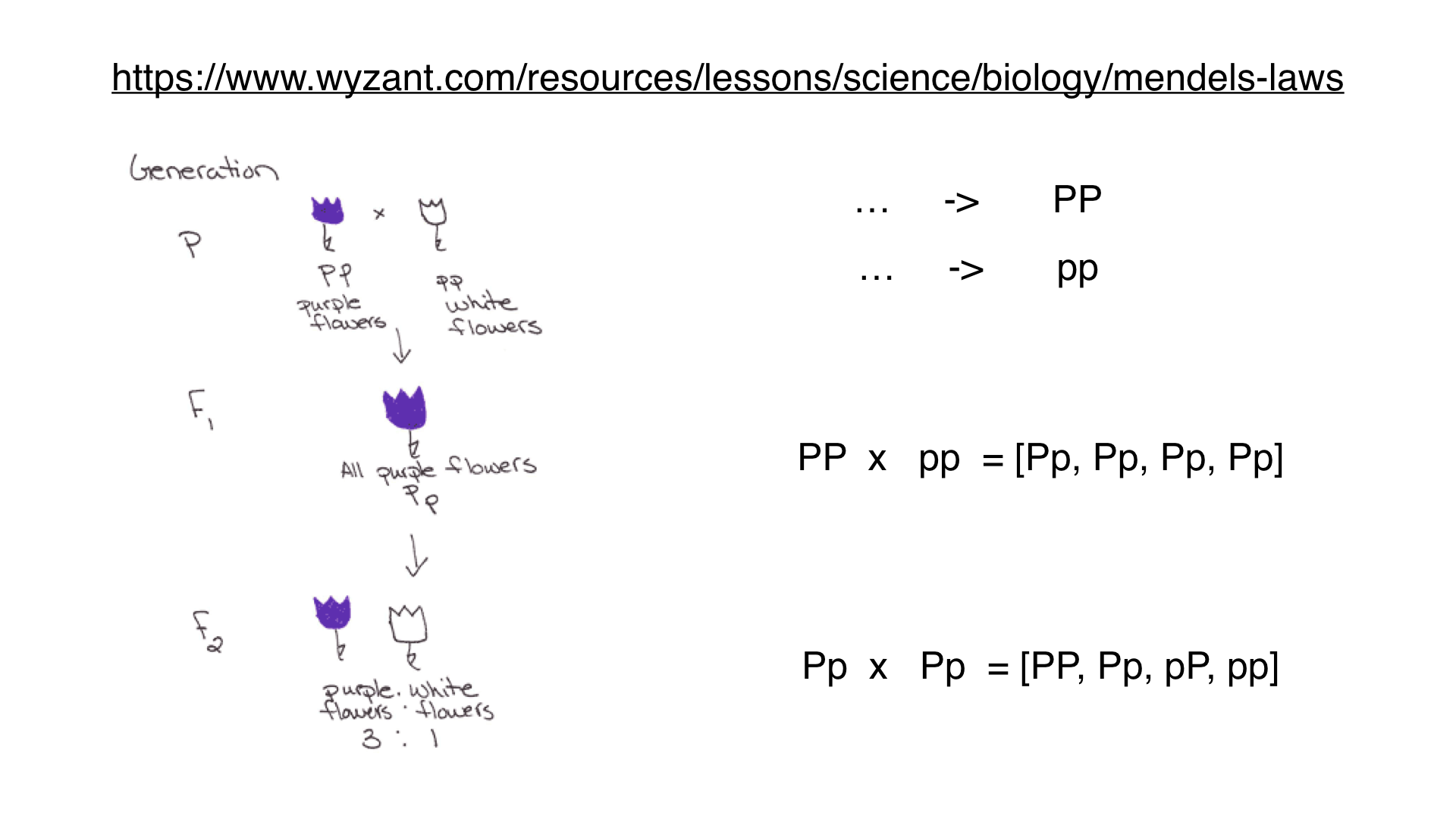In :
from collections import Counter
data=np.random.choice(['purple','purple','purple','white'],100)
Counter(data)['purple']
Out:
74
In :
results200=[]
for _ in xrange(10000):
data=np.random.choice(['purple','purple','purple','white'],200)
results200.append(Counter(data)['purple']/200.)
In :
results800=[]
for _ in xrange(10000):  #10000 simulations
data=np.random.choice(['purple','purple','purple','white'],800)
results800.append(Counter(data)['purple']/800.)
In :
plt.hist(results200,bins=np.arange(.5,1.0001,.01),color='darkblue',alpha=.7)
plt.hist(results800,bins=np.arange(.5,1.0001,.01),color='gold',alpha=.7)
plt.xlim(.65,.85);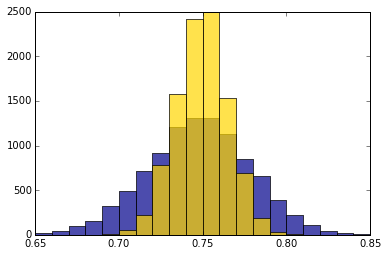In :
#expect
print np.std(results200),np.std(results800)
print 'expected',np.sqrt(.25*.75/200), np.sqrt(.25*.75/800)
0.0305588739608 0.0154937950302
expected 0.0306186217848 0.0153093108924
In [ ]:

For $m$ successes in $N$ trials:

Standard deviation of number count = $\sqrt{Np(1-p)}$

The fraction of successes is $m/N$

Standard deviation of the fraction of successes is therefore: $\sqrt{Np(1-p)}/N= \sqrt{p(1-p)/N}$

(The $\sqrt N$ is now in the denominator)

For $N=200$ trials, we expect a number count of

$Np \pm \sqrt{Np(1-p)} = 200*.75\pm \sqrt{200\cdot.25\cdot.75} =150 \pm 6.12$,

and as a percentage we expect

$p \pm \sqrt{p(1-p)/N} = .75 \pm \sqrt{.25\cdot.75/200} = .75\pm .03$,

or for $N=800$ trials, we expect number count of

$Np \pm \sqrt{Np(1-p)} = 800*.75\pm \sqrt{800\cdot.25\cdot.75} =600 \pm 12.24$,

and as a percentage we expect

$p \pm \sqrt{p(1-p)/N} = .75 \pm \sqrt{.25\cdot.75/200} = .75\pm .015$,

In [ ]:

Airline departure and arrival delay data¶

https://www.rita.dot.gov/bts/sites/rita.dot.gov.bts/files/subject_areas/airline_information/index.html

"YEAR","DEP_DELAY_NEW","ARR_DELAY",
2016,0.00,-19.00,
2016,0.00,-12.00,
2016,0.00,-15.00,
2016,0.00,-22.00,
2016,0.00,-1.00,
2016,0.00,-11.00,
2016,0.00,-5.00,
...

In :
import gzip
delays=[line.split(',')[1:3] for line in gzip.open('delays/938298838_T_ONTIME.csv.gz')
if line.startswith('2016')]
In :
dep,arr=zip(*delays)
dep=[float(m) for m in dep if m]  #convert to float if non-empty
arr=[float(m) for m in arr if m]
print len(arr),len(dep)
print 'departures:',np.mean(dep),'min on avg, std=',np.std(dep)
print 'arrivals:',np.mean(arr),'min on avg, std=',np.std(arr)
452229 453722
departures: 15.7628812356 min on avg, std= 46.5023260036
arrivals: 8.6647340175 min on avg, std= 49.8136191515

wait ... average departure delays are 15.76 min, but average arrival delays are only 8.66 min?
seems like flight schedules systematically overestimate flight times by an average of 7 min (or could just be that early departures are counted as zero delay).

Have a look at min and max times arrival delays:

In :
print 'arrival delays'
print 'from {} (= {}hr {}min)'.format(int(min(arr)),int(min(arr)/60),int(min(arr))%60)
print 'to {} (= {}hr {}min)'.format(int(max(arr)),int(max(arr)/60),int(max(arr))%60)
arrival delays
from -73 (= -1hr 47min)
to 2028 (= 33hr 48min)
In :
#the z-values are quite large
z_arr=(sorted(arr)-np.mean(arr))/np.std(arr)
z_arr[-10:]
Out:
array([ 26.60588186,  27.50924926,  27.56947376,  27.83044656,
28.71373914,  30.03867801,  30.0989025 ,  31.28331754,
32.80900472,  40.53781477])
In :
#percentage greater than 3 sigma
len(z_arr[z_arr>3])/float(len(arr))
#almost 2 percent, for normal would be more than 100 times smaller
Out:
0.01702677183462361

Recall the "Chebychev Bound": For all distributions, and all numbers $k$, the proportion of entries that are in the range "mean $\pm k$ SDs" is at least $1-1/k^2$.

This gives a lower bound, not an exact value or an approximation; but holds for all distributions, no matter how irregular, e.g.:
the proportion in the range "mean $\pm 2$ SDs" is at least 1 - 1/4 = 0.75
the proportion in the range "mean $\pm 3$ SDs" is at least 1 - 1/9 = 0.89
the proportion in the range "mean $\pm 4.5$ SDs" is at least 1 - 1/$4.5^2$ = 0.95

The percent of entries in the range "mean $\pm2$ SDs" might be much larger than 75%, as is the case for the normal distribution, but can never be smaller.

In :
#hist(arr,bins=arange(-100,2100,25))
plt.hist(arr,bins=np.arange(8.665-2*49.813, 2100, 49.813/2))
plt.xlabel('arrival delay, in minutes')
plt.xlim(-125,425);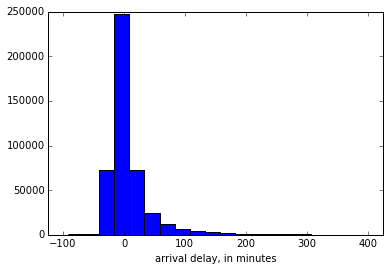In :
plt.hist(z_arr, bins=np.arange(-5,15.5,.5))
plt.xlabel('arrival delay, in std from mean of 8.66 min')
plt.xlim(-3,9);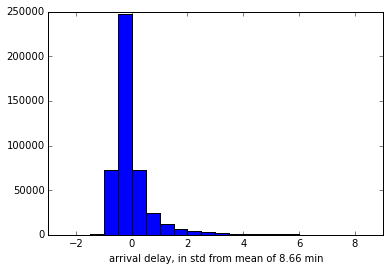In [ ]:
# to see departure delays (where early departures are counted as 0)
plt.hist(dep,bins=np.arange(-100,2100,25))
plt.xlim(-125,425);
In [ ]:
# or the z values
hist((dep-mean(dep))/std(dep), bins=arange(-5,15.5,.5))
In [ ]:

Consider a sample poll of n=1000 people, of whom k=550 answered 'Yes' to some question.

As explained above, we can consider either the standard deviation of the number count, or the standard deviation of the percentage.

The standard deviation of the number count for a Bernoulli trial consisting of n events each with probability $q$ of success was derived in class to be $\sigma=\sqrt{nq(1-q)}$. That means that if the actual probability is $q$, then we would expect to get $nq$ Yes responses, and if we keep redoing the poll with different samples of n people taken from the same distribution, then 68% of the time we would expect to get values of k between $nq-\sigma$ and $nq+\sigma$. If we do the poll only once and get k yes votes then our estimate of the underlying probability is p=k/n, but it could by off due to the finite sample size. If we keep redoing the poll many times and average the values of p, they will get closer and closer to the underlying probability $q$.

Since p=k/n, the standard deviation of the estimated probability is given by dividing the standard deviation of the number count by n: $\sqrt{np(1-p)}\ /\ n = \sqrt{p(1-p)/n}$. Notice that the n is now in the denominator under the square root. (That is because the standard deviation of the number count had a factor of n in the numerator under the square root, and when we divide by n that gets converted into a factor of n in the denominator under the squareroot: $\sqrt{n}\ /\ n = \sqrt{1/n}$.)

Here is a slightly longer explanation of how the error estimate for the number count can be turned around into an error estimate for the underlying probability distribution. Assuming the 1000 people are drawn at random from some very large population (for some details of the assumptions that go into this, see sampling), what is the likely percentage of 'Yes' voters in the full population? The idea is that the fraction p=550/1000=.55 is sampled from some much larger population whose overall fraction is some unknown value q. Each sampled person can be considered to be a Bernoulli process with probability q of success, and therefore the number of 'Yes' voters is normally distributed with mean q and standard deviation sqrt(n*q(1-q)). Say the range of q of interest is that from which we could have drawn with at least 90% probability the 550 'Yes' votes of 1000 and inferred p=.55 . We know that values of k within 1.645 standard deviations from the mean n*q will occur 90% of the time, so the range of interest is values of q such that p=k/n is within 1.645 standard deviations above or below. We don't know the real q so can't calculate its $1.645\sigma$ precisely, but q will be close enough to p that we can estimate $\sigma=$ sqrt(p(1-p)/n), and $1.645\sigma=1.645*\sqrt{.45*.55/1000}\approx.026$. (Note that the n has switched to the denominator inside the square root, because we are considering the standard deviation of k/n, hence divide the standard deviation of k by n.) The range of q that could give the inferred value of p=.55 with 90% likelihood is thus given by q between the values $.55\pm.026$, as in the simulation below.

In [ ]: